Test: Stability Analysis of Linear Control Systems- 2

# Test: Stability Analysis of Linear Control Systems- 2 - Electrical Engineering (EE)

Test Description

## 20 Questions MCQ Test GATE Electrical Engineering (EE) 2024 Mock Test Series - Test: Stability Analysis of Linear Control Systems- 2

Test: Stability Analysis of Linear Control Systems- 2 for Electrical Engineering (EE) 2023 is part of GATE Electrical Engineering (EE) 2024 Mock Test Series preparation. The Test: Stability Analysis of Linear Control Systems- 2 questions and answers have been prepared according to the Electrical Engineering (EE) exam syllabus.The Test: Stability Analysis of Linear Control Systems- 2 MCQs are made for Electrical Engineering (EE) 2023 Exam. Find important definitions, questions, notes, meanings, examples, exercises, MCQs and online tests for Test: Stability Analysis of Linear Control Systems- 2 below.
Solutions of Test: Stability Analysis of Linear Control Systems- 2 questions in English are available as part of our GATE Electrical Engineering (EE) 2024 Mock Test Series for Electrical Engineering (EE) & Test: Stability Analysis of Linear Control Systems- 2 solutions in Hindi for GATE Electrical Engineering (EE) 2024 Mock Test Series course. Download more important topics, notes, lectures and mock test series for Electrical Engineering (EE) Exam by signing up for free. Attempt Test: Stability Analysis of Linear Control Systems- 2 | 20 questions in 60 minutes | Mock test for Electrical Engineering (EE) preparation | Free important questions MCQ to study GATE Electrical Engineering (EE) 2024 Mock Test Series for Electrical Engineering (EE) Exam | Download free PDF with solutions
 1 Crore+ students have signed up on EduRev. Have you?
Test: Stability Analysis of Linear Control Systems- 2 - Question 1

### Which of the following is the correct statement? A minimum phase network is one whose transfer function has

Test: Stability Analysis of Linear Control Systems- 2 - Question 2

### For a discrete-time system to be unstable, all the poles of the z-transfer function should lie

Detailed Solution for Test: Stability Analysis of Linear Control Systems- 2 - Question 2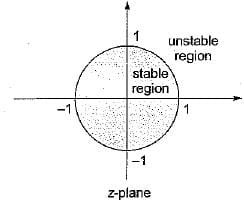Test: Stability Analysis of Linear Control Systems- 2 - Question 3

### Consider the following characteristic equation of a system: s3 + 2Ks2 + (K+ 2) s+ 4 = 0 Which one of the following is correct?

Detailed Solution for Test: Stability Analysis of Linear Control Systems- 2 - Question 3

Given characteristic equation is,
s2 + 2Ks2 + (K + 2)s + 4 = 0
Routh’s array is: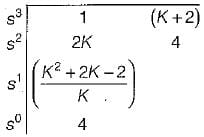For stability, 2K > 0 or K > 0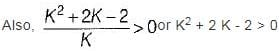Now, K2 + 2K - 2 = 0 or, K = 0.73, -2.73
For K > 0.73, K2 + 2 K - 2 > 0
(since K should be > 0)
Hence, system will be stable if K > 0.73.

Test: Stability Analysis of Linear Control Systems- 2 - Question 4

For which of the following values of K, the feedback system shown in the below figure is stable?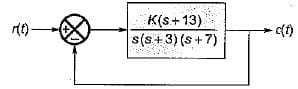Detailed Solution for Test: Stability Analysis of Linear Control Systems- 2 - Question 4

The characteristic equation is
1 + G (s) H (s) = 0
or,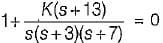or, s3 + 10s2 + (21 + K )s + 13 K= 0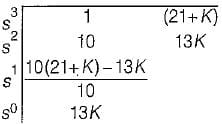For stability, 13K > 0 or K > 0
Also,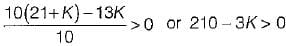or, K < 70
Hence, 0 < K< 70 (For stability).

Test: Stability Analysis of Linear Control Systems- 2 - Question 5

What is the range of K for which the open loop transfer function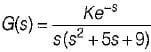represents an unstable closed loop system?

Detailed Solution for Test: Stability Analysis of Linear Control Systems- 2 - Question 5

For low frequency, e-s = (1 - s)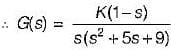The characteristic equation is
1 + G(s) = 0
or, s(s2 + 5s + 9) + K(1 - s) = 0
or, s3 + 5s2 + (9 - K) s + K = 0
The Routh’s array is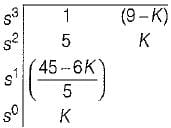For stability, K > 0 and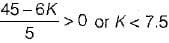∴ 0 < K < 7.5 (for stability)
0 > K > 7.5 (for unstability)

Test: Stability Analysis of Linear Control Systems- 2 - Question 6

What is the range of values of K (K > 0) such that the characteristic equation
s3 + 3 (K + 1)s2 + (7K + 5)s + (4K + 7) = 0
has roots more negative than s = -1?

Detailed Solution for Test: Stability Analysis of Linear Control Systems- 2 - Question 6

Putting s = (z - 1) in the given characteristic equation, we get:
(z - 1)3 + 3 (K + 1) (z - 1)2 + (7K+ 5) (z - 1) + (4K + 7) = 0
or, z3 + 3 Kz2 + (K + 2)z + 4 = 0
Routh’s array is: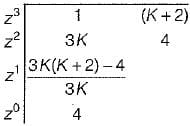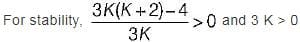i.e. 3K2 + 6K - 4 > 0 and K > 0
Now, roots of 3K2 + 6K - 4 = 0 are: K = -2.5, 0.53
Since K > 0 ∴ K = 0.53 (K = -2.5 neglected)
For K > 0.53, 3 K2 + 6 K - 4 > 0
Hence, the range of value of K is
0.53 < K < ∞ or ∞ > K > 0.53

Test: Stability Analysis of Linear Control Systems- 2 - Question 7

A closed loop control system is shown below: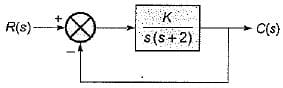Match List - I (Different values of K) with List - II (Roots of Characteristic Equation) and select the correct answer using the codes given below the lists: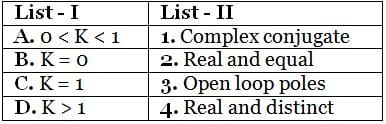Codes: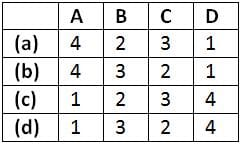Detailed Solution for Test: Stability Analysis of Linear Control Systems- 2 - Question 7

The characteristic equation is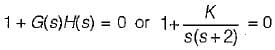or, s2 + 2 s + K = 0
or,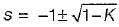When, 0 < K < 1, roots are real and distinct.
When, K = 0, roots are s = 0, -2 (Poles of open loopT.F. G(s)H(s)).
When, K = 1, roots are s = -1, -1 i.e. real and equal.
When, K > 1, roots are complex conjugate.

Test: Stability Analysis of Linear Control Systems- 2 - Question 8

Consider the following statements regarding the characteristic equation of a system given by:
s4 + 5s3 + 25+10 = 0
1. The system is unstable.
2. The system is stable.
3. Number of roots with zero real part - 0
4. Number of roots with positive real part - 4
5. Number of roots with negative real part = 2
Which of the above statements are correct?

Detailed Solution for Test: Stability Analysis of Linear Control Systems- 2 - Question 8

The Routh’s array is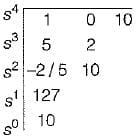Since there are two sign changes in first column of Routh’s array, therefore the system is unstable.
Number of roots with positive real part = 2
Number of roots with negative real part = 2
Number of roots with zero real part = 0

Test: Stability Analysis of Linear Control Systems- 2 - Question 9

The transfer function of a system is (1 + 2s)/(1 - s)
The system is then which one of the following?

Detailed Solution for Test: Stability Analysis of Linear Control Systems- 2 - Question 9

Since one pole (s = 1) lies in RH s-plane, therefore the system is unstable.

Test: Stability Analysis of Linear Control Systems- 2 - Question 10

Assertion (A): For a system to be stable, the roots of the characteristic equation should not lie in the right half of the s-plane or on the imaginary axis.
Reason (R): A system is said to be stable if the zeros of the closed loop transfer function are all in the left half of s-plane.

Detailed Solution for Test: Stability Analysis of Linear Control Systems- 2 - Question 10

Poles of the closed loop transfer function is the characteristic equation of a closed loop control system. For the system to be stable all roots of characteristic equation should lie in LH s-plane. Hence reason is false.

Test: Stability Analysis of Linear Control Systems- 2 - Question 11

Assertion (A): Location of the poles of a transfer function in the s-plane affects greatly the transient response of the system and hence stability.
Reason (R): The poles that are close to the imaginary axis in the left-half s-plane give rise to transient responses that will decay relatively slowly, whereas the poles that are far away from the axis (relative to the dominant poles) correspond to fast-decaying time responses.

Test: Stability Analysis of Linear Control Systems- 2 - Question 12

Assertion (A): Routh-Hurwitz criterion doesn’t provides the information about the number of roots that lie on the jω-axis while it tests whether any of the roots of the characteristic equation lie in the right-half s-plane or not.
Reason (R): The Routh-Hurwitz criterion represents a method of determining the location of zeros of a polynomial with constant real coefficients with respect to the left half and right half of the s-plane, without actually solving for the zeros.

Detailed Solution for Test: Stability Analysis of Linear Control Systems- 2 - Question 12

Routh-Hurwitz criterion tests whether any roots of the characteristic equation lie in the right-half s-plane as well as the number of roots that lie on the jω-axis.. Therefore, assertion is false.

Test: Stability Analysis of Linear Control Systems- 2 - Question 13

Consider the following statements related to Routh-Hurwitz criterion of determining the stability of a system:
1. It is applicable if the characteristic equation has real coefficients, complex terms or exponential functions of s.
2. The criterion can be applied to any stability boundaries in complex plane, such as the unit circle in the z-plane.
3. The number of changes of signs in the elements of the first column equals the number of roots with negative real parts.
Which of these statements is/are not correct?

Detailed Solution for Test: Stability Analysis of Linear Control Systems- 2 - Question 13

• Routh-Hurwitz criterion is not applicable if characteristic equation has coefficients which are exponential or complex.
• It is not applied in z-plane because z-plane having unit circle is the stability boundary of discrete-data system.
• Statement-3 is also false because no. of sign change in 1st column of Routh’s array indicates the number of roots with positive real part or roots lying in RH s-plane.

Test: Stability Analysis of Linear Control Systems- 2 - Question 14

The characteristic equation of a linear discrete-data control system is given as:
F(z) = z3 + z2 + z + K = 0,
Where K is a real constant.
What is the range of values of K so that the system is stable?

Detailed Solution for Test: Stability Analysis of Linear Control Systems- 2 - Question 14

Given, F(z) = z3 + z2 + z + k = 0
Using bilinear transformation, we put z =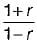in the above characteristic equation.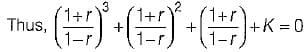or, (1 - K)r3 + (1 + 3K) r2 + 3(1 - K)r + 3 + K = 0
Routh’s array is: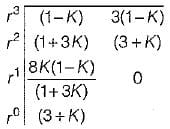For the system to be stable, we have:
1 - K > 0 or K < 1    ...(i)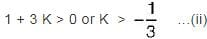3 + K > 0 or K > - 3    ..(iii)
From equation (i), (ii) and (iii) the range of values of K for stability is, 0 < K < 1.

Test: Stability Analysis of Linear Control Systems- 2 - Question 15

The characteristic equation of a linear time-invariant discrete-data system is given by:
F(z) = anzn + an - 1 zn - 1 + .......... + az + a0 = 0
where all the coefficients are real.
According to “Jury’s stability criterion”, there are certain necessary conditions which must be satisfied for the above discrete-data system to have no roots on or outside the unit circle.
Which of the following necessary condition does not holds true for the discrete-data system to have all roots inside the unit circle according to “Jury's stability criterion"?

Detailed Solution for Test: Stability Analysis of Linear Control Systems- 2 - Question 15

The necessary conditions for stability are: F (1) > 0
F(-1) > 0, if n = even integer
F(-1) < 0, if n = odd integer
and |a0| < |an|

Test: Stability Analysis of Linear Control Systems- 2 - Question 16

The first two rows of Routh’s tabulation of a third-order system are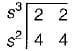Select the correct answer from the following choices.

Detailed Solution for Test: Stability Analysis of Linear Control Systems- 2 - Question 16

Routh’s array is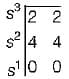Since Routh’s array ends abruptly, therefore we construct auxiliary equation for finding other elements of Routh’s array.
A2(s) = 4s2 + 4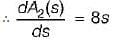Hence, new Routh’s array is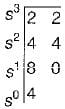Since there is no sign change in 1st column of Routh’s array, therefore given system will be marginally stable provided there is no repeated poles on jω - axis.
Thus, A2(s) = 0 or 4s2 + 4 = 0
or, s = ± j (distinct imaginary roots)
Hence, the given system is marginally stable having one root in LH s-plane and two roots on the jω - axis at s = ± j.

Test: Stability Analysis of Linear Control Systems- 2 - Question 17

The characteristic equation of a second order discrete-data system is given by:
F(z) = z2+ z+ 0.25 = 0
The above system is

Detailed Solution for Test: Stability Analysis of Linear Control Systems- 2 - Question 17

For a second order discrete- data system given by:
F(z) = a2z2 + a1z + a0 = 0
to be stable, the necessary and sufficient conditions are:
F(1) > 0
F(-1) > 0 and |a0| < a2
Here, F(z) = z2 + z + 0.25
So,  a0 = 0.25, a1 = 1, a2 = 1
Thus, F(1) - 12+ 1 + 0.25 = 2.25 > 0
F(-1) = 1 -1 + 0.25 = 0.25 > 0
and la0l = 0.25 < a2 = 1
Since ail the conditions are satisfied, therefore given system is stable.

Test: Stability Analysis of Linear Control Systems- 2 - Question 18

The z-transform of a signal is given by: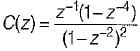Its final value is

Detailed Solution for Test: Stability Analysis of Linear Control Systems- 2 - Question 18

Given,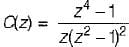Applying final value theorem,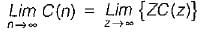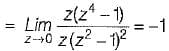Test: Stability Analysis of Linear Control Systems- 2 - Question 19

The dynamic response of a system is given by the following differential equation: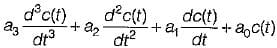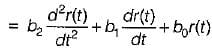where c(t) and r(t) are respectively the output and the input and a, a, a1 a0, b2, b1 and b0 are constants. It is a

Test: Stability Analysis of Linear Control Systems- 2 - Question 20

The transfer function of a system is: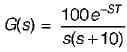The system

## GATE Electrical Engineering (EE) 2024 Mock Test Series

23 docs|285 tests
Information about Test: Stability Analysis of Linear Control Systems- 2 Page
In this test you can find the Exam questions for Test: Stability Analysis of Linear Control Systems- 2 solved & explained in the simplest way possible. Besides giving Questions and answers for Test: Stability Analysis of Linear Control Systems- 2, EduRev gives you an ample number of Online tests for practice

## GATE Electrical Engineering (EE) 2024 Mock Test Series

23 docs|285 tests